Example Sinusoids Next  |  Prev  |  Up  |  Top  |  Index  |  JOS Index  |  JOS Pubs  |  JOS Home  |  Search

### Example Sinusoids

Figure 4.1 plots the sinusoid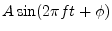, for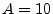,,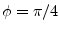, and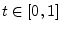. Study the plot to make sure you understand the effect of changing each parameter (amplitude, frequency, phase), and also note the definitions of peak-to-peak amplitude'' and zero crossings.''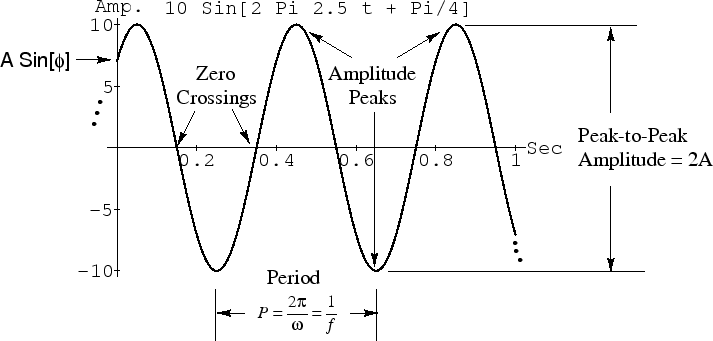A tuning fork'' vibrates approximately sinusoidally. An A-440'' tuning fork oscillates at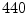cycles per second. As a result, a tone recorded from an ideal A-440 tuning fork is a sinusoid at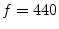Hz. The amplitude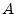determines how loud it is and depends on how hard we strike the tuning fork. The phase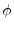is set by exactly when we strike the tuning fork (and on our choice of when time 0 is). If we record an A-440 tuning fork on an analog tape recorder, the electrical signal recorded on tape is of the form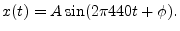As another example, the sinusoid at amplitude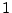and phase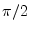(90 degrees) is simply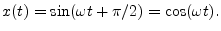Thus,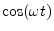is a sinusoid at phase 90-degrees, while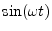is a sinusoid at zero phase. Note, however, that we could just as well have definedto be the zero-phase sinusoid rather than. It really doesn't matter, except to be consistent in any given usage. The concept of a sinusoidal signal'' is simply that it is equal to a sine or cosine function at some amplitude, frequency, and phase. It does not matter whether we choose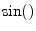or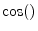in the official'' definition of a sinusoid. You may encounter both definitions. Usingis nice since sinusoid'' naturally generalizes. However, usingis nicer when defining a sinusoid to be the real part of a complex sinusoid (which we'll talk about in §4.3.11).

Next  |  Prev  |  Up  |  Top  |  Index  |  JOS Index  |  JOS Pubs  |  JOS Home  |  Search

[How to cite this work] [Order a printed hardcopy]